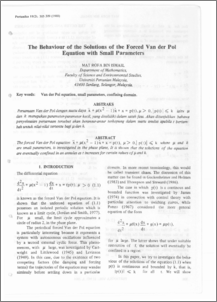# The behaviour of the solutions of the forced Van der Pol equation with small parameters

## Citation

Ismail, Mat Rofa (1988) The behaviour of the solutions of the forced Van der Pol equation with small parameters. Pertanika, 11 (2). pp. 305-309. ISSN 0126-6128

## Abstract

The forced Van der Pol equation x+ u.(x 2 - l)x + x = p(t), jJ. > 0,1 p(t)1 ~ k where u and k are small parameters, is investigated in the phase plane, It is shown that the solutions of the equation are eventually confined in an annulas as t increases for certain values of u and kPreview
PDF
The_Behaviour_of_the_Solutions_of_the_Forced_Van_der_Pol.pdfView Item